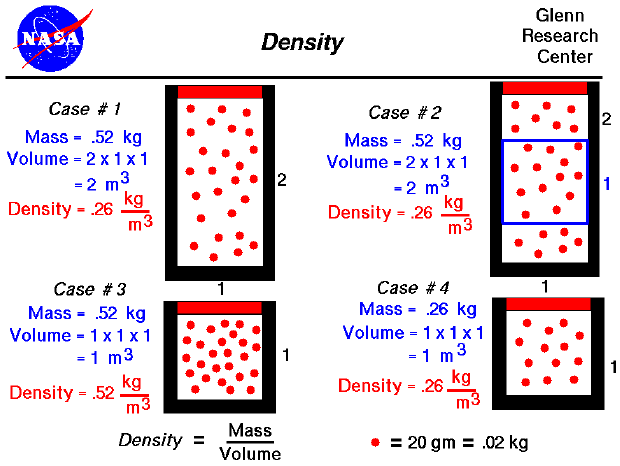An important property of any gas (including air) is density. Density is defined as the mass of an object divided by its volume, and most of our experiences with density involve solids. We know that some objects are heavier than other objects, even though they are the same size. A brick and a loaf of bread are about the same size, but a brick is heavier--it is more dense. Among metals, aluminum is less dense than iron. That's why airplanes and some automobile parts are made from aluminum. For the same volume of material, one metal weighs less than another.

For solids, because the molecules are bound to one another, the density of a single element or compound remains fairly constant. For example, if you found a pure gold nugget on the earth or you found a pure gold nugget on the moon, the measured density would be nearly the same. But for gases, because the molecules are free to move about, density can vary over a wide range. Air at the surface of the earth has a very different density than air 50 kilometers above the earth. An interactive simulator allows you to study how air density varies with altitude. Understanding density and how it works is fundamental to the understanding of aerodynamics.

From the kinetic theory of gases, a gas is composed of a large number of molecules that are very small relative to the distance between molecules. The molecules are in constant, random motion and frequently collide with each other and with the walls of a container. Because the molecules are in motion, a gas will expand to fill the container. Since density is defined to be the mass divided by the volume, density depends directly on the size of the container in which a gas is confined. As a simple example, consider Case #1 on our figure. We have 26 molecules of a mythical gas. Each molecule has a mass of 20 grams (.02 kilograms), so the mass of this gas is .52 kg. We have confined this gas in a rectangular tube that is 1 meter on each side and 2 meters high. We are viewing the tube from the front, so the dimension into the slide is 1 meter for all the cases considered. The volume of the tube is 2 cubic meters, so the density is .26 kg/cubic meter. (This corresponds to air at about 13 kilometers altitude.) If the size of our container were decreased to 1 meter on all sides, as in Case #3, and we kept the same number of molecules, that density would increase to .52 kg/cubic meter. (Notice that we have the same amount of material; it is just contained in a smaller volume. How we decrease the volume is very important for the final value of pressure and temperature. You can explore the variations in pressure and temperature at the animated gas lab).

Actual molecules of a gas are incredibly small. In one cubic meter the number of molecules is about ten to the 23rd power. (That's 1 followed by 23 zero's !!!) For a static gas, the molecules are in a completely random motion. Because there are so many molecules, and the motion of each molecule is random, the value of the density is the same throughout the container. As an example, consider Case #1, in which the mass is .52 kg, the volume is 2 cu m, and the density is .26 kg/cu m. If we sample a smaller volume of 1 meter on a side as in Case #2, we will obtain the same density. The volume of the blue box in Case #2 is only 1 cu m, but the number of molecules in the box is 13 at .2 kg per molecule; and the density is .26 kg/cu m. (This example REALLY works only for a very large number of molecules moving at random. Case #2 is just an illustration.) Another way to obtain the same density for a smaller volume is to remove molecules from the container. In Case #4, the container is the same size as in Case #2, but the number of molecules (the mass) has been decreased to only 13 molecules. The density is .26 kg/cubic meter, which is the same density seen in the blue box of Case #2 and throughout Case #1. A careful study of these four cases will help you understand the meaning of gas density.

These rather simple examples help explain a fundamental effect that we see in nature. Between Cases #3 and #4, the number of molecules in a given volume decreased, and the corresponding density decreased. In the atmosphere, air molecules near the surface of the earth are held together more tightly than the molecules in a higher atmosphere because of the gravitational pull of the earth on all the molecules above the surface molecules. The higher up you go in the atmosphere, the fewer the molecules there are above you, and the lower the confining force. So in the atmosphere, density decreases as you increase altitude; there are fewer molecules.Guided Tours

Beginner's Guide to Aerodynamics
Beginner's Guide to Propulsion
Beginner's Guide to Model Rockets
Beginner's Guide to Kites
Beginner's Guide to Aeronautics